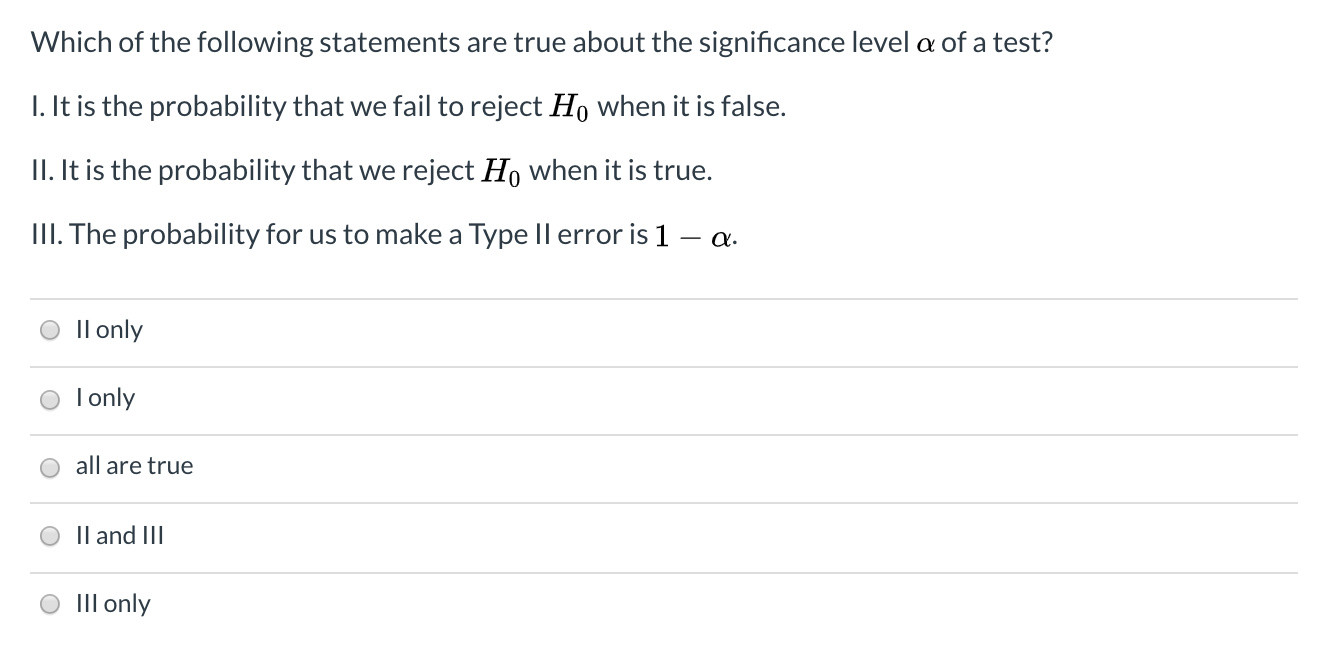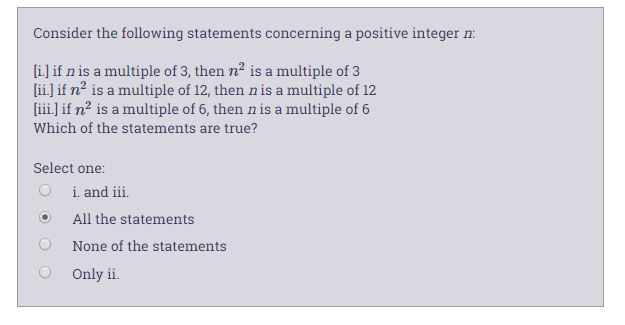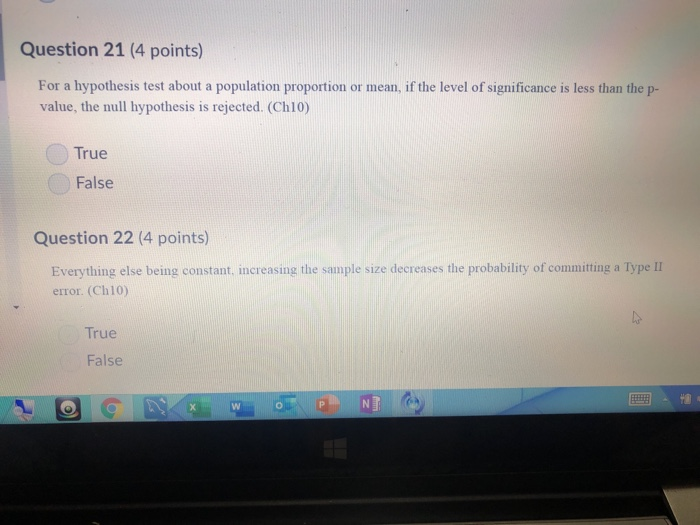Question

Consider the following four statements concerning hypothesis tests. I. We can decrease the probability of a Type II error by increasing the sample size. II. We can decrease the probability of a Type II error by decreasing the sample size. III. We can decrease the probability of a Type I error by increasing the level of significance . IV. We can decrease the probability of a Type I error by decreasing the level of significance . Which statements above are true?

(a) I only

(b) II only

(c) I and IV only

(d) II and III only

(e) None

Option (c) 1 and 4 only

(1) We can decrease the probability of a Type II error by increasing the sample size.

(4) We can decrease the probability of a Type I error by decreasing the level of significance .

#### Earn Coins

Coins can be redeemed for fabulous gifts.

Similar Homework Help Questions
• ### 1. Which of the following statements are not generally true? a. A type I error is...

1. Which of the following statements are not generally true? a. A type I error is usually more serious than a type II error. b. A type II error is usually more serious than a type I error. c. A test with significance level  is one for which the type I error probability is controlled at the specified level. d. When an experiment and a sample size are fixed, then decreasing the size of the rejection region to obtain...

• ### Which of the following statements are true about the significance level a of a test? 1. It is the probability that we f...Which of the following statements are true about the significance level a of a test? 1. It is the probability that we fail to reject Ho when it is false. II. It is the probability that we reject Ho when it is true. III. The probability for us to make a Type Il error is 1 - a. O ll only o Tonly O all are true O II and III O III only

• ### Consider the following statements concerning a positive integer : (i.) if n is a multiple of...Consider the following statements concerning a positive integer : (i.) if n is a multiple of 3, then n2 is a multiple of 3 [ii.) if n' is a multiple of 12, then n is a multiple of 12 (iii.) if nis a multiple of 6, then nis a multiple of 6 Which of the statements are true? Select one: O i. and iii. O All the statements None of the statements Only ii.

• ### Which of the following statements is FALSE? a.) The power of a hypothesis test is the...

Which of the following statements is FALSE? a.) The power of a hypothesis test is the probability of not making a Type II error. b.) Alpha (α) is equal to the probability of making a Type I error. c.) The probability of rejecting the null hypothesis when the null hypothesis is true is called a Type I Error. d.) A smaller sample size would increase the effectiveness of a hypothesis test.

• ### α is the probability of a Type I error, which occurs when we accept the alternative...

α is the probability of a Type I error, which occurs when we accept the alternative H1 when the null hypothesis Ho is true. True False A Type II error occurs when when a false null hypothesis is rejected. True False If a null hypothesis is rejected at the 5% significance level but not at the 1% significance level, then the p-value of the test is less than 1%. True False The power of a test is the probability of...

• ### say a student uses their personalized class data set to test the hypothesis that more than...

say a student uses their personalized class data set to test the hypothesis that more than 50% of the people in class are in Chemistry, and rejects the null hypothesis at the 2% significance level. Consider the following statements. (i) The proportion of students in this class who are in Life Sciences is in fact greater than 50%. (ii) The p-value is greater than .02. (iii) The probability of Type II error is less than than 2%. (iv) A Type...

• ### hypothesis testing

supposethe mean score of students taking the preparatory course is 522.Was a type I or type II error committed?if we tested this hypothesis at theα=0.01 level, what is the probability of committing a type I error?and b----if we wanted to decrease the probability of making type II error, would beneed toincrease or decrease the level of significance?

• ### Question 21 (4 points) For a hypothesis test about a population proportion or mean, if the...Question 21 (4 points) For a hypothesis test about a population proportion or mean, if the level of significance is less than the p- value, the null hypothesis is rejected. (Ch10) True False Question 22 (4 points) Everything else being constant, increasing the sample size decreases the probability of committing a Type II error. (Ch10) True False The power of a statistical test is the probability of not rejecting the null hypothesis when it is true. (Ch10) True False Question...

• ### State whether the following statements true or false, and Why? 1. The type I error and...

State whether the following statements true or false, and Why? 1. The type I error and type II error are related. A decrease in the probability of one generally results in an increase in the probability of the other. 2. The size of the critical region, and therefore the probability of committing a type I error, can always be reduced by adjusting the critical value(s). 3. An increase in the sample size n will reduce α and β simultaneously. 4....

• ### Which of the following statements is true about the P-value? A. It is the probability of...

Which of the following statements is true about the P-value? A. It is the probability of committing type I error and it is the smallest significant level at which a null hypothesis would be rejected B. It is the probability of committing type I error and it is the largest significant level at which a null hypothesis would be rejected C. It is the probability of committing type II error and it is the smallest significant level at which a...